## Section 3: Hierarchal modeling¶

A key strength of Bayesian modeling is the easy and flexibility with which one can implement a hierarchical model. This section will implement and compare a pooled & partially pooled model.

In :
import itertools
import matplotlib.pyplot as plt
import numpy as np
import pandas as pd
import pymc3 as pm
import scipy
import scipy.stats as stats
import seaborn.apionly as sns

from IPython.display import Image
from sklearn import preprocessing

%matplotlib inline
plt.style.use('bmh')
colors = ['#348ABD', '#A60628', '#7A68A6', '#467821', '#D55E00',
'#CC79A7', '#56B4E9', '#009E73', '#F0E442', '#0072B2']



### Model Pooling¶

Let's explore a different way of modeling the response time for my hangout conversations. My intuition would suggest that my tendency to reply quickly to a chat depends on who I'm talking to. I might be more likely to respond quickly to my girlfriend than to a distant friend. As such, I could decide to model each conversation independently, estimating parameters $\mu_i$ and $\alpha_i$ for each conversation $i$.

One consideration we must make, is that some conversations have very few messages compared to others. As such, our estimates of response time for conversations with few messages will have a higher degree of uncertainty than conversations with a large number of messages. The below plot illustrates the discrepancy in sample size per conversation.

In :
ax = messages.groupby('prev_sender')['conversation_id'].size().plot(
kind='bar', figsize=(12,3), title='Number of messages sent per recipient', color=colors)
_ = ax.set_xlabel('Previous Sender')
_ = ax.set_ylabel('Number of messages')
_ = plt.xticks(rotation=45)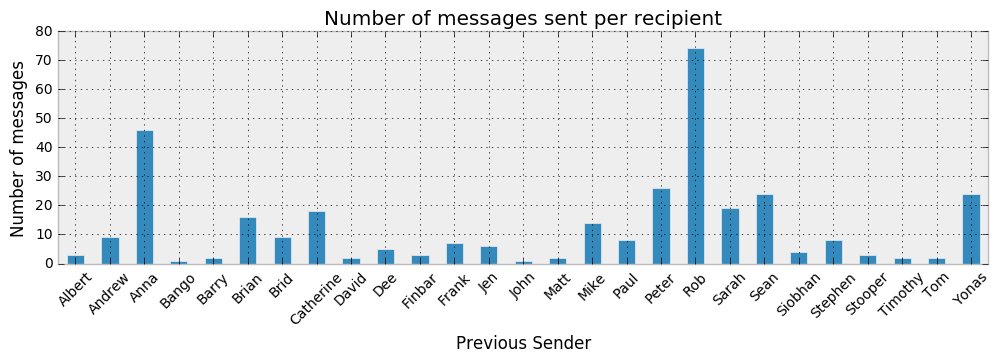For each message j and each conversation i, we represent the model as:

$$y_{ji} \sim NegBinomial(\mu_i, \alpha_i)$$$$\mu_i = Uniform(0, 100)$$$$\alpha_i = Uniform(0, 100)$$
In :
indiv_traces = {}

# Convert categorical variables to integer
le = preprocessing.LabelEncoder()
participants_idx = le.fit_transform(messages['prev_sender'])
participants = le.classes_
n_participants = len(participants)

for p in participants:
with pm.Model() as model:
alpha = pm.Uniform('alpha', lower=0, upper=100)
mu = pm.Uniform('mu', lower=0, upper=100)

data = messages[messages['prev_sender']==p]['time_delay_seconds'].values
y_est = pm.NegativeBinomial('y_est', mu=mu, alpha=alpha, observed=data)

y_pred = pm.NegativeBinomial('y_pred', mu=mu, alpha=alpha)

start = pm.find_MAP()
step = pm.Metropolis()
trace = pm.sample(20000, step, start=start, progressbar=True)

indiv_traces[p] = trace

Applied interval-transform to alpha and added transformed alpha_interval_ to model.
Applied interval-transform to mu and added transformed mu_interval_ to model.
100%|██████████| 20000/20000 [00:09<00:00, 2042.61it/s]
Applied interval-transform to alpha and added transformed alpha_interval_ to model.
Applied interval-transform to mu and added transformed mu_interval_ to model.
100%|██████████| 20000/20000 [00:09<00:00, 2001.80it/s]
Applied interval-transform to alpha and added transformed alpha_interval_ to model.
Applied interval-transform to mu and added transformed mu_interval_ to model.
100%|██████████| 20000/20000 [00:10<00:00, 1903.35it/s]
Applied interval-transform to alpha and added transformed alpha_interval_ to model.
Applied interval-transform to mu and added transformed mu_interval_ to model.
100%|██████████| 20000/20000 [00:08<00:00, 2444.60it/s]
Applied interval-transform to alpha and added transformed alpha_interval_ to model.
Applied interval-transform to mu and added transformed mu_interval_ to model.
100%|██████████| 20000/20000 [00:10<00:00, 1894.24it/s]
Applied interval-transform to alpha and added transformed alpha_interval_ to model.
Applied interval-transform to mu and added transformed mu_interval_ to model.
100%|██████████| 20000/20000 [00:10<00:00, 1875.85it/s]
Applied interval-transform to alpha and added transformed alpha_interval_ to model.
Applied interval-transform to mu and added transformed mu_interval_ to model.
100%|██████████| 20000/20000 [00:09<00:00, 2072.87it/s]
Applied interval-transform to alpha and added transformed alpha_interval_ to model.
Applied interval-transform to mu and added transformed mu_interval_ to model.
100%|██████████| 20000/20000 [00:10<00:00, 1890.48it/s]
Applied interval-transform to alpha and added transformed alpha_interval_ to model.
Applied interval-transform to mu and added transformed mu_interval_ to model.
100%|██████████| 20000/20000 [00:09<00:00, 2041.61it/s]
Applied interval-transform to alpha and added transformed alpha_interval_ to model.
Applied interval-transform to mu and added transformed mu_interval_ to model.
100%|██████████| 20000/20000 [00:09<00:00, 2085.20it/s]
Applied interval-transform to alpha and added transformed alpha_interval_ to model.
Applied interval-transform to mu and added transformed mu_interval_ to model.
100%|██████████| 20000/20000 [00:09<00:00, 2078.25it/s]
Applied interval-transform to alpha and added transformed alpha_interval_ to model.
Applied interval-transform to mu and added transformed mu_interval_ to model.
100%|██████████| 20000/20000 [00:10<00:00, 1879.97it/s]
Applied interval-transform to alpha and added transformed alpha_interval_ to model.
Applied interval-transform to mu and added transformed mu_interval_ to model.
100%|██████████| 20000/20000 [00:09<00:00, 2074.33it/s]
Applied interval-transform to alpha and added transformed alpha_interval_ to model.
Applied interval-transform to mu and added transformed mu_interval_ to model.
100%|██████████| 20000/20000 [00:07<00:00, 2605.87it/s]
Applied interval-transform to alpha and added transformed alpha_interval_ to model.
Applied interval-transform to mu and added transformed mu_interval_ to model.
100%|██████████| 20000/20000 [00:10<00:00, 1880.07it/s]
Applied interval-transform to alpha and added transformed alpha_interval_ to model.
Applied interval-transform to mu and added transformed mu_interval_ to model.
100%|██████████| 20000/20000 [00:10<00:00, 1969.21it/s]
Applied interval-transform to alpha and added transformed alpha_interval_ to model.
Applied interval-transform to mu and added transformed mu_interval_ to model.
100%|██████████| 20000/20000 [00:10<00:00, 1824.27it/s]
Applied interval-transform to alpha and added transformed alpha_interval_ to model.
Applied interval-transform to mu and added transformed mu_interval_ to model.
100%|██████████| 20000/20000 [00:10<00:00, 1969.02it/s]
Applied interval-transform to alpha and added transformed alpha_interval_ to model.
Applied interval-transform to mu and added transformed mu_interval_ to model.
100%|██████████| 20000/20000 [00:09<00:00, 2042.10it/s]
Applied interval-transform to alpha and added transformed alpha_interval_ to model.
Applied interval-transform to mu and added transformed mu_interval_ to model.
100%|██████████| 20000/20000 [00:10<00:00, 1930.11it/s]
Applied interval-transform to alpha and added transformed alpha_interval_ to model.
Applied interval-transform to mu and added transformed mu_interval_ to model.
100%|██████████| 20000/20000 [00:09<00:00, 2076.93it/s]
Applied interval-transform to alpha and added transformed alpha_interval_ to model.
Applied interval-transform to mu and added transformed mu_interval_ to model.
100%|██████████| 20000/20000 [00:10<00:00, 1985.03it/s]
Applied interval-transform to alpha and added transformed alpha_interval_ to model.
Applied interval-transform to mu and added transformed mu_interval_ to model.
100%|██████████| 20000/20000 [00:10<00:00, 1848.71it/s]
Applied interval-transform to alpha and added transformed alpha_interval_ to model.
Applied interval-transform to mu and added transformed mu_interval_ to model.
100%|██████████| 20000/20000 [00:08<00:00, 2381.88it/s]
Applied interval-transform to alpha and added transformed alpha_interval_ to model.
Applied interval-transform to mu and added transformed mu_interval_ to model.
100%|██████████| 20000/20000 [00:06<00:00, 3271.07it/s]
Applied interval-transform to alpha and added transformed alpha_interval_ to model.
Applied interval-transform to mu and added transformed mu_interval_ to model.
100%|██████████| 20000/20000 [00:06<00:00, 3025.22it/s]
Applied interval-transform to alpha and added transformed alpha_interval_ to model.
Applied interval-transform to mu and added transformed mu_interval_ to model.
100%|██████████| 20000/20000 [00:06<00:00, 3014.88it/s]

In :
fig, axs = plt.subplots(3,2, figsize=(12, 6))
axs = axs.ravel()
y_left_max = 2
y_right_max = 2000
x_lim = 60
ix = [3,4,6]

for i, j, p in zip([0,1,2], [0,2,4], participants[ix]):
axs[j].set_title('Observed: %s' % p)
axs[j].hist(messages[messages['prev_sender']==p]['time_delay_seconds'].values, range=[0, x_lim], bins=x_lim, histtype='stepfilled')
axs[j].set_ylim([0, y_left_max])

for i, j, p in zip([0,1,2], [1,3,5], participants[ix]):
axs[j].set_title('Posterior predictive distribution: %s' % p)
axs[j].hist(indiv_traces[p].get_values('y_pred'), range=[0, x_lim], bins=x_lim, histtype='stepfilled', color=colors)
axs[j].set_ylim([0, y_right_max])

axs.set_xlabel('Response time (seconds)')
axs.set_xlabel('Response time (seconds)')

plt.tight_layout()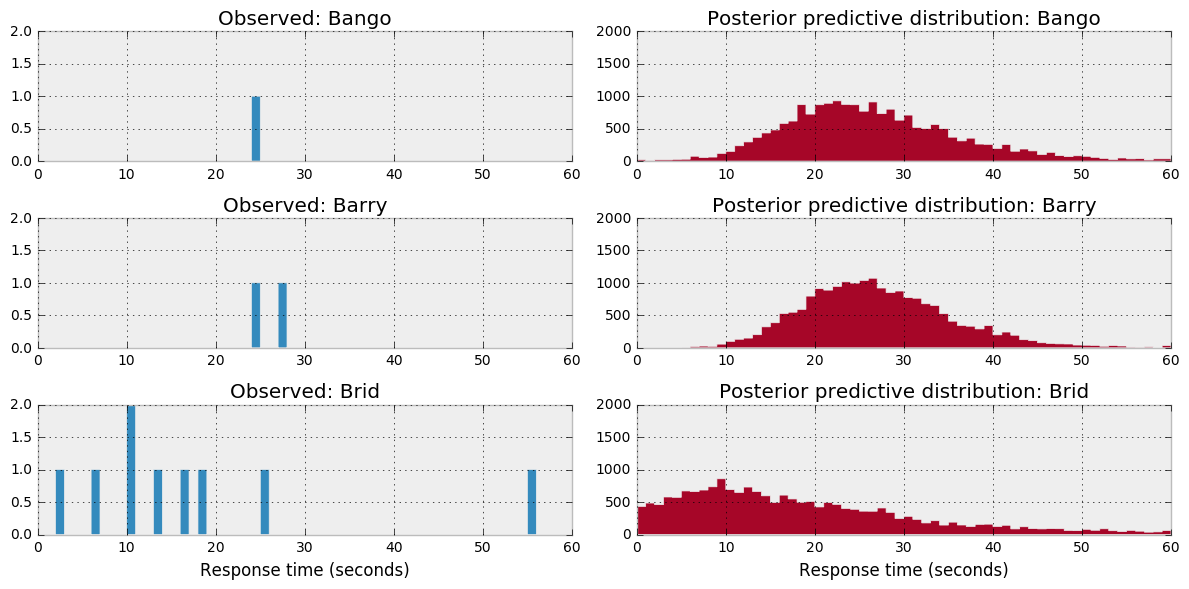The above plots show the observed data (left) and the posterior predictive distribution (right) for 3 example conversations we modeled. As you can see, the posterior predictive distribution can vary considerably across conversations. This could accurately reflect the characteristics of the conversation or it could be inaccurate due to small sample size.

If we combine the posterior predictive distributions across these models, we would expect this to resemble the distribution of the overall dataset observed. Let's perform the posterior predictive check.

In :
combined_y_pred = np.concatenate([v.get_values('y_pred') for k, v in indiv_traces.items()])

x_lim = 60
y_pred = trace.get_values('y_pred')

fig = plt.figure(figsize=(12,6))

_ = plt.hist(combined_y_pred, range=[0, x_lim], bins=x_lim, histtype='stepfilled', color=colors)
_ = plt.xlim(1, x_lim)
_ = plt.ylim(0, 20000)
_ = plt.ylabel('Frequency')
_ = plt.title('Posterior predictive distribution')

_ = plt.hist(messages['time_delay_seconds'].values, range=[0, x_lim], bins=x_lim, histtype='stepfilled')
_ = plt.xlim(0, x_lim)
_ = plt.xlabel('Response time in seconds')
_ = plt.ylim(0, 20)
_ = plt.ylabel('Frequency')
_ = plt.title('Distribution of observed data')

plt.tight_layout()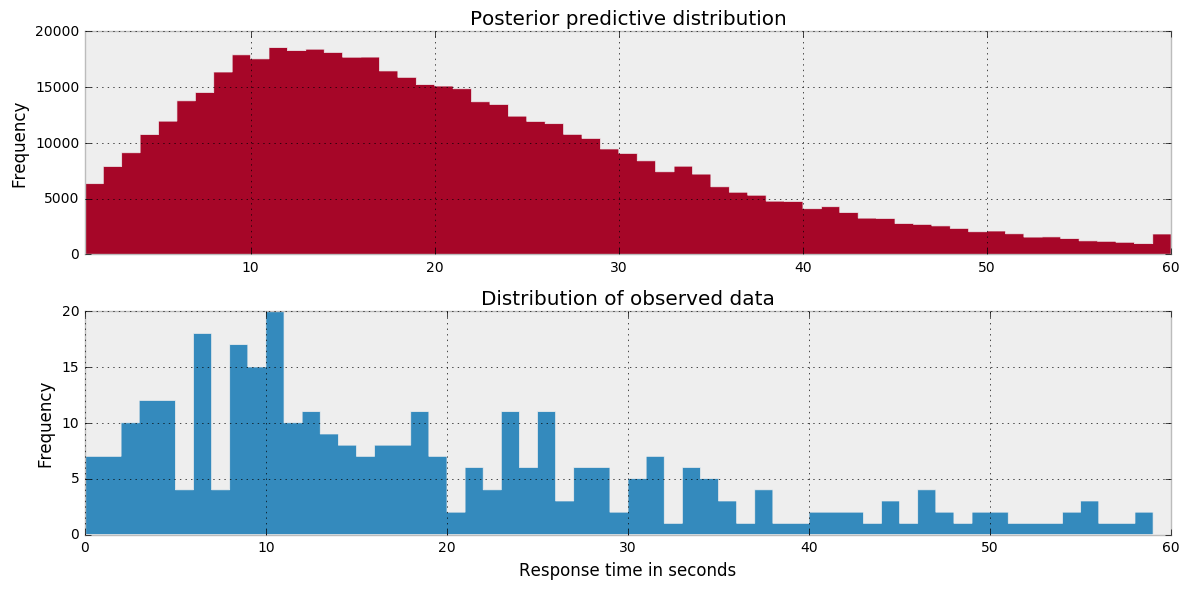Yes, the posterior predictive distribution resembles the distribution of the observed data. However, I'm concerned that some of the conversations have very little data and hence the estimates are likely to have high variance. One way to mitigate this risk to to share information across conversations - but still estimate $\mu_i$ for each conversation. We call this partial pooling.

### Partial pooling¶

Just like in the pooled model, a partially pooled model has paramater values estimated for each conversation i. However, parameters are connected together via hyperparameters. This reflects our belief that my response_time's per conversation have similarities with one another via my own natural tendancy to respond quickly or slowly.

$$y_{ji} \sim NegBinomial(\mu_i, \alpha_i)$$

Following on from the above example, we will estimate parameter values $(\mu_i)$ and $(\alpha_i)$ for a Poisson distribution. Rather than using a uniform prior, I will use a Gamma distribution for both $\mu$ and $\sigma$. This will enable me to introduce more prior knowledge into the model as I have certain expectations as to what vales $\mu$ and $\sigma$ will be.

First, let's have a look at the Gamma distribution. As you can see below, it is very flexible.

In :
mu = [5,25,50]
sd = [3,7,2]

plt.figure(figsize=(11,3))
_ = plt.title('Gamma distribution')

with pm.Model() as model:
for i, (j, k) in enumerate(zip(mu, sd)):
samples = pm.Gamma('gamma_%s' % i, mu=j, sd=k).random(size=10**6)
plt.hist(samples, bins=100, range=(0,60), color=colors[i], alpha=1)

_ = plt.legend(['$\mu$ = %s, $\sigma$ = %s' % (mu[a], sd[a]) for a in [0,1,2]])

Applied log-transform to gamma_0 and added transformed gamma_0_log_ to model.
Applied log-transform to gamma_1 and added transformed gamma_1_log_ to model.
Applied log-transform to gamma_2 and added transformed gamma_2_log_ to model.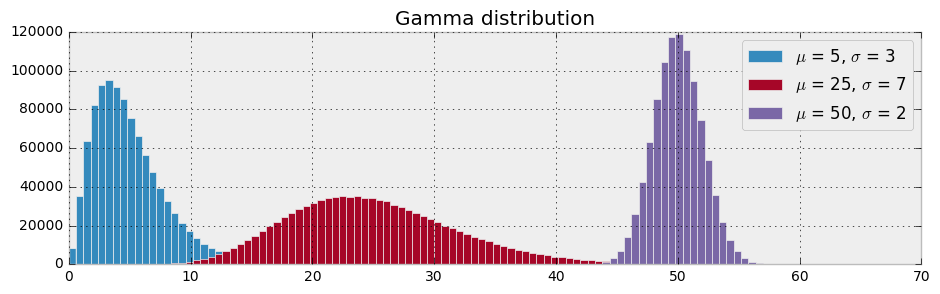The partially pooled model can be formally described by:

$$y_{ji} \sim NegBinomial(\mu_i, \alpha_i)$$$$\mu_i = Gamma(\mu_\mu, \sigma_\mu)$$$$\alpha_i = Gamma(\mu_\alpha, \sigma_\alpha)$$$$\mu_\mu = Uniform(0, 60)$$$$\sigma_\mu = Uniform(0, 50)$$$$\mu_\alpha = Uniform(0, 10)$$$$\sigma_\alpha = Uniform(0, 50)$$
In :
Image('graphics/dag neg poisson gamma hyper.png', width=420)

Out: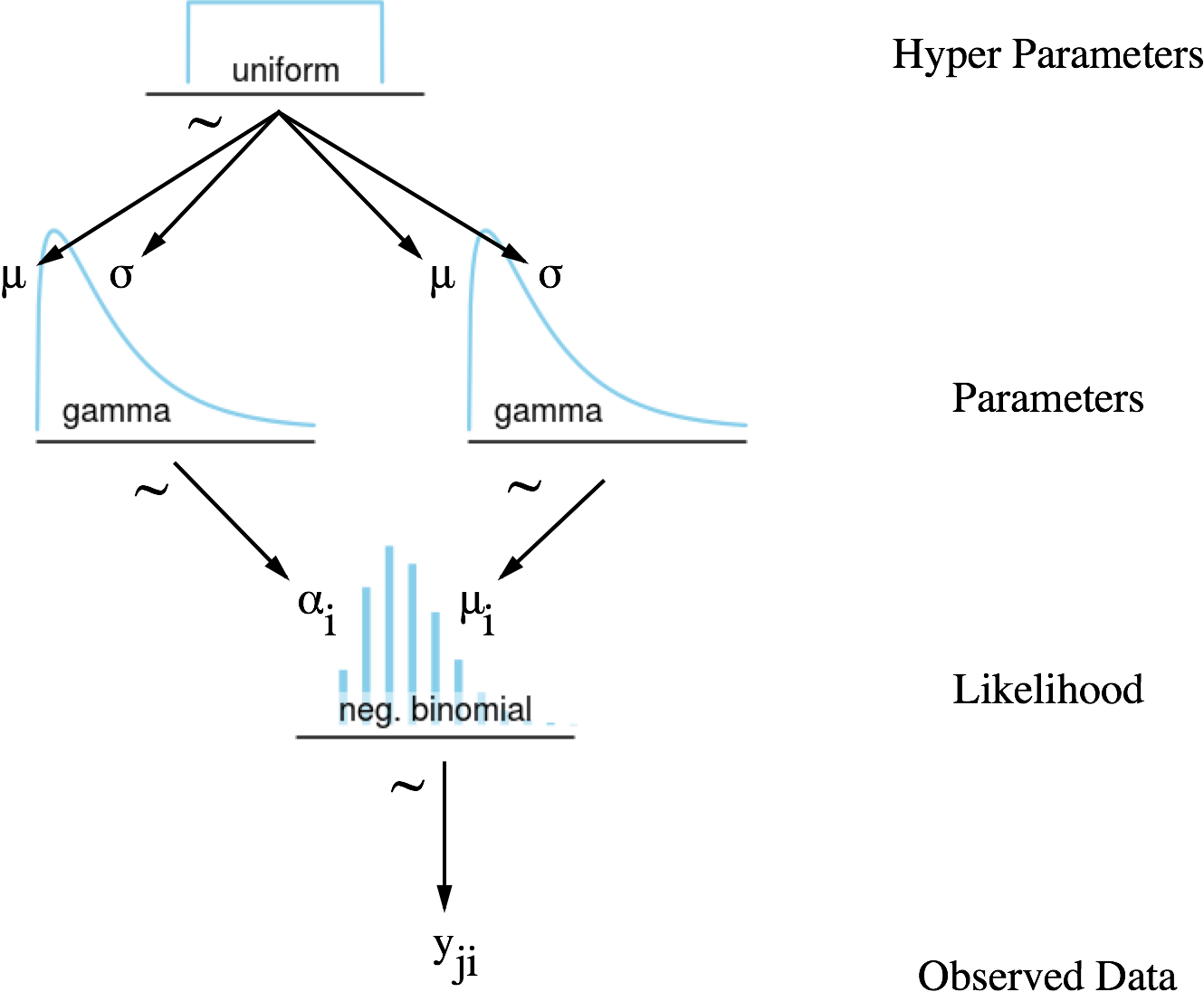In :
with pm.Model() as model:
hyper_alpha_sd = pm.Uniform('hyper_alpha_sd', lower=0, upper=50)
hyper_alpha_mu = pm.Uniform('hyper_alpha_mu', lower=0, upper=10)

hyper_mu_sd = pm.Uniform('hyper_mu_sd', lower=0, upper=50)
hyper_mu_mu = pm.Uniform('hyper_mu_mu', lower=0, upper=60)

alpha = pm.Gamma('alpha', mu=hyper_alpha_mu, sd=hyper_alpha_sd, shape=n_participants)
mu = pm.Gamma('mu', mu=hyper_mu_mu, sd=hyper_mu_sd, shape=n_participants)

y_est = pm.NegativeBinomial('y_est',
mu=mu[participants_idx],
alpha=alpha[participants_idx],
observed=messages['time_delay_seconds'].values)

y_pred = pm.NegativeBinomial('y_pred',
mu=mu[participants_idx],
alpha=alpha[participants_idx],
shape=messages['prev_sender'].shape)

start = pm.find_MAP()
step = pm.Metropolis()
hierarchical_trace = pm.sample(200000, step, progressbar=True)

Applied interval-transform to hyper_alpha_sd and added transformed hyper_alpha_sd_interval_ to model.
Applied interval-transform to hyper_alpha_mu and added transformed hyper_alpha_mu_interval_ to model.
Applied interval-transform to hyper_mu_sd and added transformed hyper_mu_sd_interval_ to model.
Applied interval-transform to hyper_mu_mu and added transformed hyper_mu_mu_interval_ to model.
Applied log-transform to alpha and added transformed alpha_log_ to model.
Applied log-transform to mu and added transformed mu_log_ to model.
100%|██████████| 200000/200000 [03:55<00:00, 848.81it/s]

In :
_ = pm.traceplot(hierarchical_trace[120000:],
varnames=['mu','alpha','hyper_mu_mu',
'hyper_mu_sd','hyper_alpha_mu',
'hyper_alpha_sd'])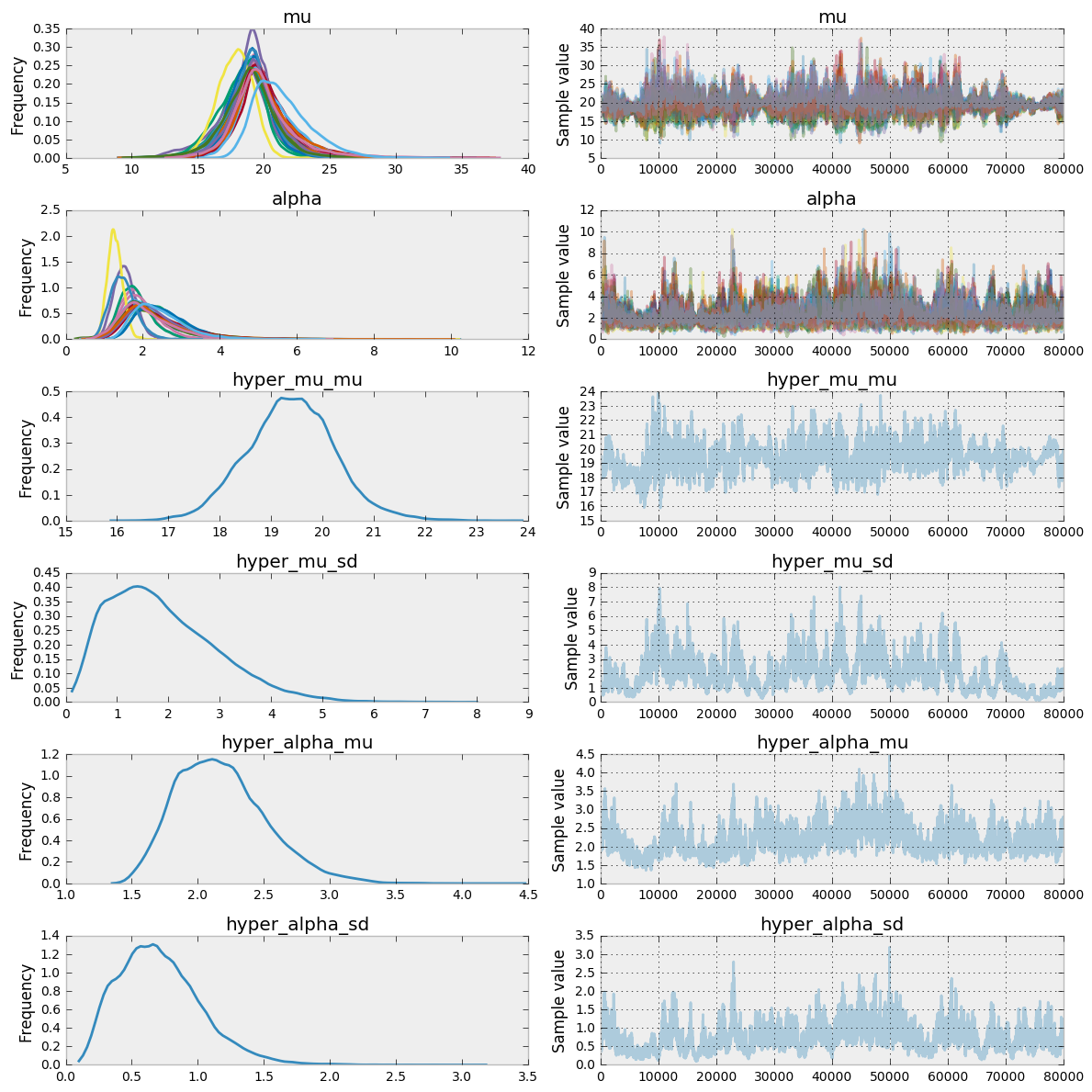You can see for the estimates of $\mu$ and $\alpha$ that we have multiple plots - one for each conversation i. The difference between the pooled and the partially pooled model is that the parameters of the partially pooled model ($\mu_i$ and $\alpha_i$) have a hyperparameter that is shared across all conversations i. This brings two benefits:

1. Information is shared across conversations, so for conversations that have limited sample size, they "borrow" knowledge from other conversations during estimation to help reduce the variance of the estimate
2. We get an estimate for each conversation and an overall estimate for all conversations

Let's have a quick look at the posterior predictive distribution.

In :
x_lim = 60
y_pred = hierarchical_trace.get_values('y_pred')[::1000].ravel()

fig = plt.figure(figsize=(12,6))

_ = plt.hist(y_pred, range=[0, x_lim], bins=x_lim, histtype='stepfilled', color=colors)
_ = plt.xlim(1, x_lim)
_ = plt.ylabel('Frequency')
_ = plt.title('Posterior predictive distribution')

_ = plt.hist(messages['time_delay_seconds'].values, range=[0, x_lim], bins=x_lim, histtype='stepfilled')
_ = plt.xlabel('Response time in seconds')
_ = plt.ylabel('Frequency')
_ = plt.title('Distribution of observed data')

plt.tight_layout()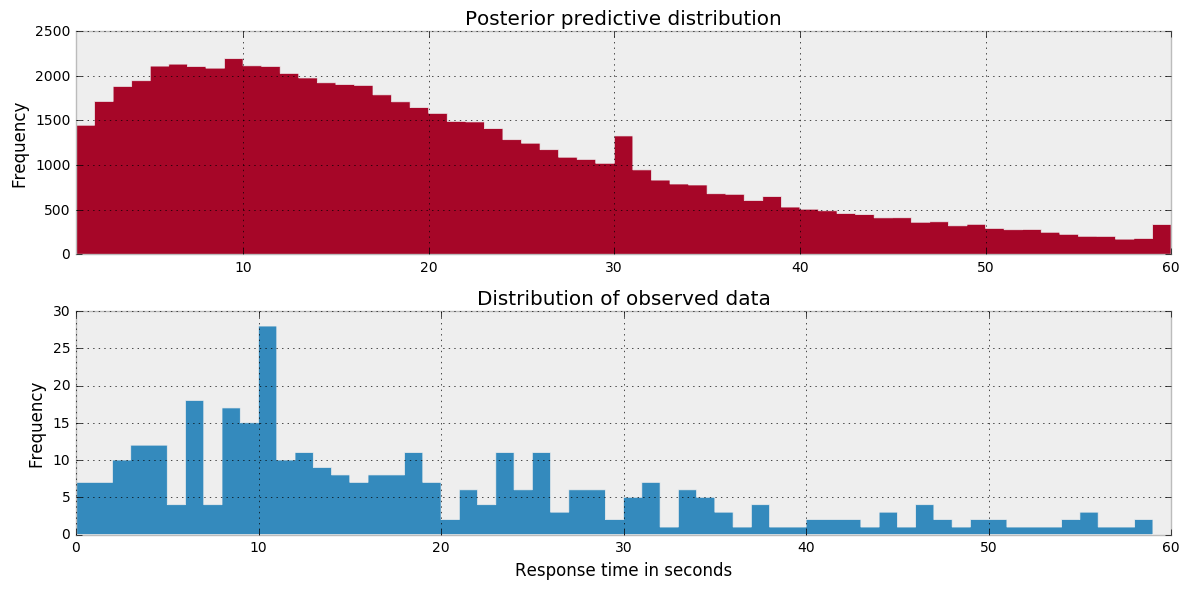### Shrinkage effect: pooled vs hierarchical model¶

As discussed, the partially pooled model shared a hyperparameter for both $\mu$ and $\alpha$. By sharing knowledge across conversations, it has the effect of shrinking the estimates closer together - particularly for conversations that have little data.

This shrinkage effect is illustrated in the below plot. You can see how the $\mu$ and $\alpha$ parameters are drawn together by the effect of the hyperparameter.

In :
hier_mu = hierarchical_trace['mu'][500:].mean(axis=0)
hier_alpha = hierarchical_trace['alpha'][500:].mean(axis=0)
indv_mu = [indiv_traces[p]['mu'][500:].mean() for p in participants]
indv_alpha = [indiv_traces[p]['alpha'][500:].mean() for p in participants]

fig = plt.figure(figsize=(8, 6))
title='Pooled vs. Partially Pooled Negative Binomial Model',
xlim=(5, 45), ylim=(0, 10))

ax.scatter(indv_mu, indv_alpha, c=colors, s=50, label = 'Pooled', zorder=3)
ax.scatter(hier_mu, hier_alpha, c=colors, s=50, label = 'Partially Pooled', zorder=4)
for i in range(len(indv_mu)):
ax.arrow(indv_mu[i], indv_alpha[i], hier_mu[i] - indv_mu[i], hier_alpha[i] - indv_alpha[i],

_ = ax.legend()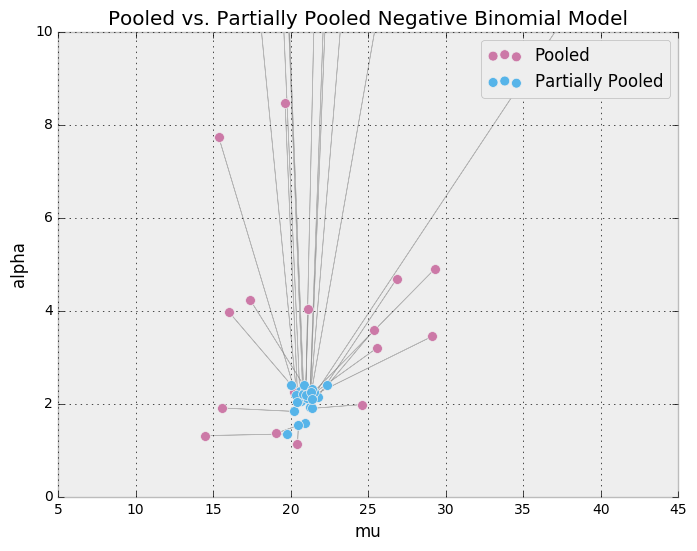### Asking questions of the posterior¶

Let's start to take advantage of one of the best aspects of Bayesian statistics - the posterior distribution. Unlike frequentist techniques, we get a full posterior distribution as opposed to a single point estimate. In essence, we have a basket full of credible parameter values. This enables us to ask some questions in a fairly natural and intuitive manner.

#### What are the chances I'll respond to my friend in less than 10 seconds?¶

To estimate this probability, we can look at the posterior predctive distribution for Timothy & Andrew's response_time and check how many of the samples are < 10 seconds. When I first heard of this technique, I thought I misunderstood because it seemed overly simplistic.

In :
def participant_y_pred(person):
"""Return posterior predictive for person"""
ix = np.where(participants == person)
return hierarchical_trace['y_pred'][100000:, ix]

In :
print("Here are some samples from Timothy's posterior predictive distribution: \n %s" % participant_y_pred('Yonas'))

Here are some samples from Timothy's posterior predictive distribution:
[17 17 17 ..., 16 16 16]

In :
def person_plotA(person_name):
ix_check = participant_y_pred(person_name) > 10
_ = plt.hist(participant_y_pred(person_name)[~ix_check], range=[0, x_lim], bins=x_lim, histtype='stepfilled', label='<10 seconds')
_ = plt.hist(participant_y_pred(person_name)[ix_check], range=[0, x_lim], bins=x_lim, histtype='stepfilled', label='>10 seconds')
_ = plt.title